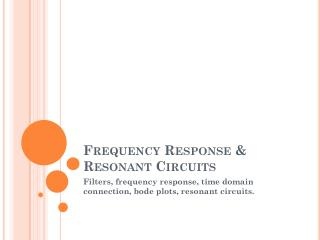# Frequency Response & Resonant Circuits - PowerPoint PPT PresentationDownload PresentationFrequency Response & Resonant Circuits

Frequency Response & Resonant CircuitsDownload Presentation## Frequency Response & Resonant Circuits

- - - - - - - - - - - - - - - - - - - - - - - - - - - E N D - - - - - - - - - - - - - - - - - - - - - - - - - - -
##### Presentation Transcript

1. Frequency Response & Resonant Circuits Filters, frequency response, time domain connection, bode plots, resonant circuits.

2. Outline and topics Reading Boylestad Ch 21.1-21.11 Boylestad Ch 20.1-20.8 • Low-pass filters • High-pass filters • Other filters • Resonance (Ch 20) • Ideal op-amps and active filters • Decibels & log scales • Linear systems and transfer functions • Bode plots

3. Filters

4. FILTERS • Any combination of passive (R, L, and C) and/or active (transistors or operational amplifiers) elements designed to select or reject a band of frequencies is called a filter. • In communication systems, filters are used to pass those frequencies containing the desired information and to reject the remaining frequencies.

5. FILTERS • In general, there are two classifications of filters: • Passive filters-gain always<1 • Active filters-gain can be >1

6. FIG. 21.9 R-C low-pass filter at low frequencies. FIG. 21.8 Low-pass filter. R-C LOW-PASS FILTER

7. FIG. 21.10 R-C low-pass filter at high frequencies. FIG. 21.11 Vo versus frequency for a low-pass R-C filter. R-C LOW-PASS FILTER

8. FIG. 21.12 Normalized plot of Fig. 21.11. R-C LOW-PASS FILTER

9. FIG. 21.13 Angle by which Vo leads Vi. R-C LOW-PASS FILTER

10. FIG. 21.14 Angle by which Vo lags Vi. R-C LOW-PASS FILTER

11. FIG. 21.15 Low-pass R-L filter. FIG. 21.16 Example 21.5. R-C LOW-PASS FILTER

12. FIG. 21.17 Frequency response for the low-pass R-C network in Fig. 21.16. R-C LOW-PASS FILTER

13. FIG. 21.18 Normalized plot of Fig. 21.17. R-C LOW-PASS FILTER

14. FIG. 21.19 High-pass filter. R-C HIGH-PASS FILTER

15. FIG. 21.20 R-C high-pass filter at very high frequencies. FIG. 21.21 R-C high-pass filter at f =0 Hz. R-C HIGH-PASS FILTER

16. FIG. 21.22 Vo versus frequency for a high-pass R-C filter. R-C HIGH-PASS FILTER

17. FIG. 21.23 Normalized plot of Fig. 21.22. R-C HIGH-PASS FILTER

18. FIG. 21.24 Phase-angle response for the high-pass R-C filter. R-C HIGH-PASS FILTER

19. FIG. 21.25 High-pass R-L filter. R-C HIGH-PASS FILTER

20. FIG. 21.26 Normalized plots for a low-pass and a high-pass filter using the same elements. R-C HIGH-PASS FILTER

21. FIG. 21.27 Phase plots for a low-pass and a high-pass filter using the same elements. R-C HIGH-PASS FILTER

22. FIG. 21.28 Series resonant pass-band filter. PASS-BAND FILTERS

23. RLC Circuits-resonance! • The resonant electrical circuit must have both inductance and capacitance. • In addition, resistance will always be present due either to the lack of ideal elements or to the control offered on the shape of the resonance curve. • When resonance occurs due to the application of the proper frequency ( fr), the energy absorbed by one reactive element is the same as that released by another reactive element within the system.

24. SERIES RESONANT CIRCUIT • A resonant circuit (series or parallel) must have an inductive and a capacitive element. • A resistive element is always present due to the internal resistance of the source (Rs), the internal resistance of the inductor (Rl), and any added resistance to control the shape of the response curve (Rdesign).

25. FIG. 20.2 Series resonant circuit. SERIES RESONANT CIRCUIT

26. FIG. 21.29 Parallel resonant pass-band filter. PASS-BAND FILTERS

27. FIG. 21.30 Series resonant pass-band filter for Example 21.7. PASS-BAND FILTERS

28. FIG. 21.31 Pass-band response for the network. PASS-BAND FILTERS

29. FIG. 21.32 Normalized plots for the pass-band filter in Fig. 21.30. PASS-BAND FILTERS

30. FIG. 21.33 Pass-band filter. PASS-BAND FILTERS

31. FIG. 21.34 Pass-band characteristics. PASS-BAND FILTERS

32. FIG. 21.36 Pass-band characteristics for the filter in Fig. 21.35. FIG. 21.35 Pass-band filter. PASS-BAND FILTERS

33. FIG. 21.37 Network of Fig. 21.35 at f =994.72 kHz. PASS-BAND FILTERS

34. BAND-REJECT FILTERS • Since the characteristics of a band-reject filter (also called stop-band or notch filter) are the inverse of the pattern obtained for the band-pass filter, a band-reject filter can be designed by simply applying Kirchhoff’s voltage law to each circuit.

35. FIG. 21.38 Demonstrating how an applied signal of fixed magnitude can be broken down into a pass-band and band-reject response curve. BAND-REJECT FILTERS

36. FIG. 21.39 Band-reject filter using a series resonant circuit. BAND-REJECT FILTERS

37. FIG. 21.40 Band-reject filter using a parallel resonant network. BAND-REJECT FILTERS

38. FIG. 21.41 Band-reject filter. BAND-REJECT FILTERS

39. FIG. 21.42 Band-reject characteristics. BAND-REJECT FILTERS

40. Operational amplifiers Active filters

41. amplifiers give gain • Simple amp-1 input and 1 output • Gain, A=Vout/Vin

42. Example • If the amplifier above gives an output voltage of 1000V with an input voltage of 50V, what is the gain?

43. ideal operational-amplifier(op-amp) http://www.youtube.com/watch?v=TQB1VlLBgJE • Inputs draw no current-infinite input impedace • Vout=A(Vplus-Vminus) A-open loop gain. • A is ideally infinity-How is this useful? • Output can provide as much voltage/current as needed-zero output impedance

44. negative feedback • Negative feedback (NF) tries to reduce the difference • with NF, Vplus=Vminus ALWAYS • summing point constraints • virtual ground.

45. Inverting amplifier • Input goes into Vminus input-INVERTING input • Gain, Ainv=-R2/R1, gain is negative because inverting

46. inverting amplifier • Vplus=Vminus • Inputs draw no current

47. Non-inverting amplifier • Input goes into Vplus input-NON-INVERTING input • Gain, Ainv=1+R2/R1, gain is positive

48. unity gain buffer • Gain is 1 i.e. Vin=Vout • Used to isolate one side from the other

49. Real op-amps http://www.national.com/mpf/LM/LM324.html#Overview • Output voltage determined by rails (power supply) • Open loop gain not infinity • Inputs draw small amount of current-nA’s or less Quad LM324 Single LM741